News

# New Low-Power, Zero-Drift Operational Amplifier from Texas Instruments, the OPA2333P

November 29, 2018 by Dr. Steve Arar

## This article will take a look at the TI’s OPA2333P which is a micro-power high-precision operational amplifier.

This article will take a look at the TI’s OPA2333P which is a micro-power high-precision operational amplifier.

The OPA2333P operates from a single or dual-supply 1.8 V to 5.5 V source, has a maximum offset voltage of 10 $$\mu V$$, and incorporates a zero-drift structure which yields a maximum offset voltage drift of 0.05 $$\mu V/ ^{\circ} C$$. The zero-drift feature also improves the noise performance of the device at low frequencies.

The OPA2333P exhibits 1.1 $$\mu V_{P-P}$$ noise from 0.01 Hz to 10 Hz. The device has rail-to-rail inputs and outputs and draws a quiescent current of 17 $$\mu A$$. All of this is packed into a 2mm x 2mm WSON package.

### Functional Block Diagram

The functional block diagram of the OPA2333P is shown in Figure 1.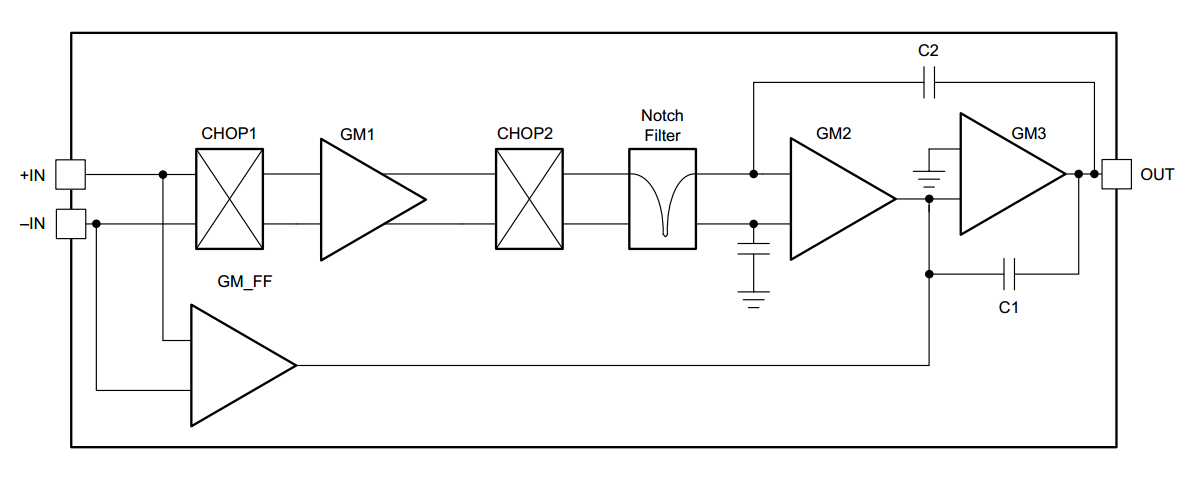##### Figure 1. Image courtesy of Texas Instruments.

The device uses a proprietary offset cancellation technique which reduces the offset voltage to less than 10 $$\mu V$$ and yields a near zero-drift operation.

There are four transconductance stages denoted by GM1, GM2, GM3, and GM_FF. The blocks represented by CHOP1 and CHOP2 are switching networks used to implement the mentioned auto-calibration technique. A notch filter is used to further attenuate errors. In the rest of the article, we’ll briefly look at the offset reduction technique employed in TI’s high-precision op-amps.

### Offset Voltage and Drift

Input offset voltage ($$V_{OS}$$) and input offset voltage drift over time or temperature ($$\frac{dV_{OS}}{dT}$$) are two important parameters of an amplifier. When amplifying a low-frequency signal, $$V_{OS}$$ of the amplifier can be indistinguishable from the desired signal being measured. This can introduce measurement errors. Besides, the offset voltage drift can make this error vary with time or temperature. Hence, the amplifier offset and drift should be carefully chosen based on the requirements of a given application.

For fairly high-resolution measurements, we often need an offset voltage below 1 mV. Having the amplifier offset and drift values and the application operating temperature range, we can find the worst case offset error over temperature by:

$$|V_{OS,max}| = |V_{OS(25^{\circ}C)}| + | \frac{dV_{OS}}{dT} | \times (Application \; Temperature \; Range)$$

This maximum offset error gives us some information about the minimum DC voltage that can be measured by the amplifier, though there are other factors, such as the amplifier noise, that can affect the minimum detectable value. You can find details and examples in this TI community article on nanopower op amps.

When working with micro-volt signals, amplifiers with very low offset voltage and drift are required. Some amplifiers, such as the OPA2333P, use an internal calibration mechanism to effectively reduce the offset voltage and drift. These amplifiers are often referred to as zero-drift amplifiers. The maximum offset and drift of the OPA2333P are 10 $$\mu V$$ and 0.05 $$\mu V/ ^{\circ} C$$, respectively.

### How a Zero-Drift Amplifier Reduces the Offset and Drift?

Let’s take a look at the technique the OPA2333P uses to perform offset cancellation. The goal is to eliminate the offset from GM1 in Figure 1. This offset voltage can be modeled as a voltage source at the input of GM1 as shown in Figures 2 and 3 below. In these figures, the switching networks represent the circuitry inside the CHOP1 and CHOP2 blocks in Figure 1.

While the input is applied to +IN and -IN pins, the offset can be modeled as a voltage source at the input of GM1. This is the main feature that allows the OPA2333P to distinguish between the input signal and the offset voltage.

The offset cancellation technique incorporates two different half cycles. The first half cycle is shown in Figure 2. The voltage $$V_{OS}$$ is amplified by Gm1 and applied to capacitor $$C_{c}$$ at the input of the next stage. The switches are configured in a way that the lower plate of $$C_{c}$$ has a positive charge.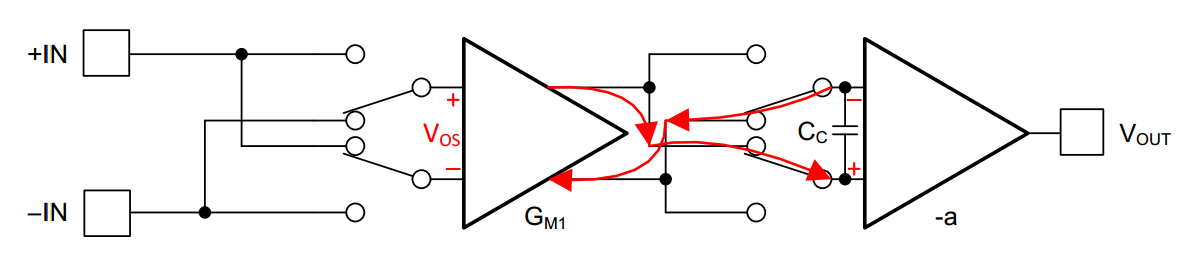##### Figure 2. Image courtesy of Texas Instruments.

Now, consider the other phase as shown in Figure 3. Again the voltage $$V_{OS}$$ experiences the gain of Gm1 and then gets sampled on $$C_{c}$$. However, in this case, the switch configuration has changed and the upper plate of the capacitor has a positive charge. In other words, the switches are arranged in a way that the offset voltage reaches GM2 without inversion in one half cycle and with inversion in the other half cycle. Hence, the error voltage produced by the offset voltage will be averaged to zero.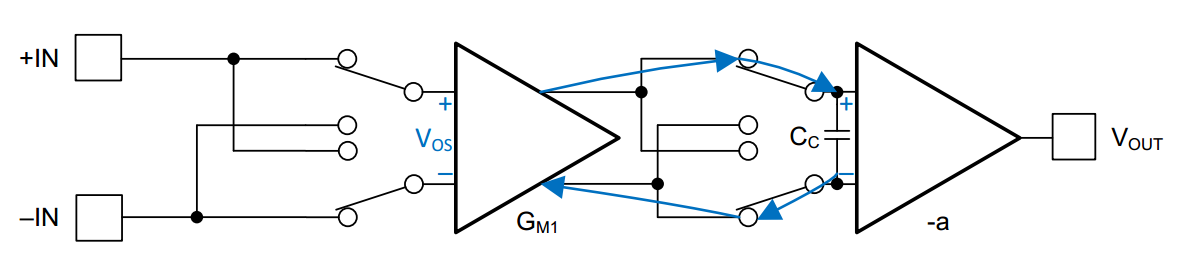##### Figure 3. Image courtesy of Texas Instruments.

To prevent the input signal from being canceled out, another set of switches are placed between the input pins and Gm1. These switches are turn on and off in a way that the input signal always reaches GM2 with the same polarity. Hence, the input voltage won’t be averaged to zero by the self-correcting mechanism.

Now let’s look at some other interesting features of the device.

### Low-Frequency Noise Performance

The self-correcting mechanism not only reduces the offset and drift but also suppresses the low-frequency noise of the device. Figure 4 below compares the low-frequency noise of a zero-drift amplifier with that of a traditional continuous-time amplifier.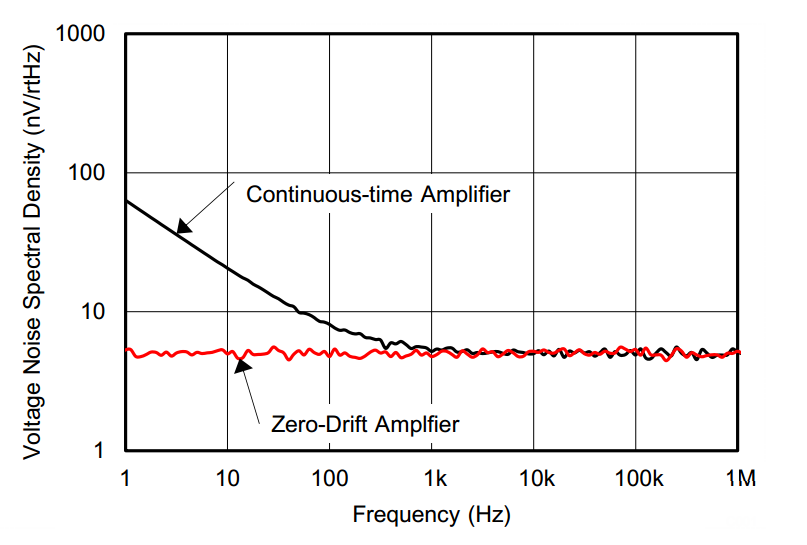##### Figure 4. Image courtesy of Texas Instruments.

Generally, the low-frequency noise of zero-drift amplifiers is much lower than a continuous-time amplifier. The OPA2333P exhibits 1.1 $$\mu V_{P-P}$$ noise from 0.01 Hz to 10 Hz.

### Specified Start-Up Time

To ensure high-precision operation, the OPA2333P incorporates a specified start-up time of 500 $$\mu s$$ after the device is powered. After the start-up time, the device is guaranteed to achieve its specified accuracy. The test configuration and the definition of the start-up time are illustrated in Figures 5 and 6, respectively.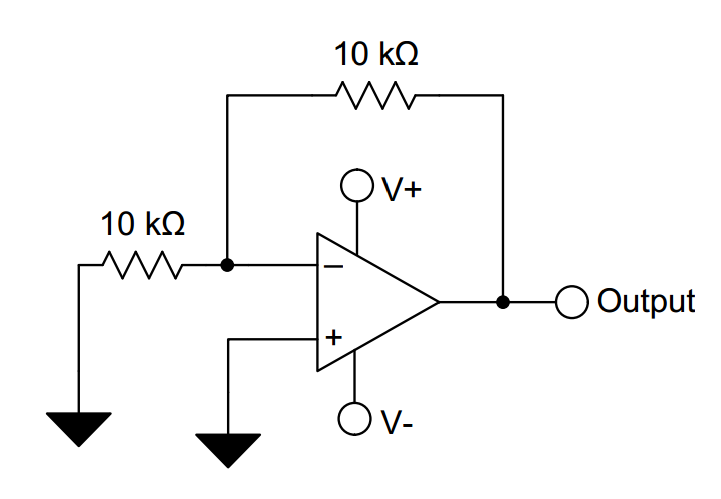##### Figure 5. Image courtesy of Texas Instruments.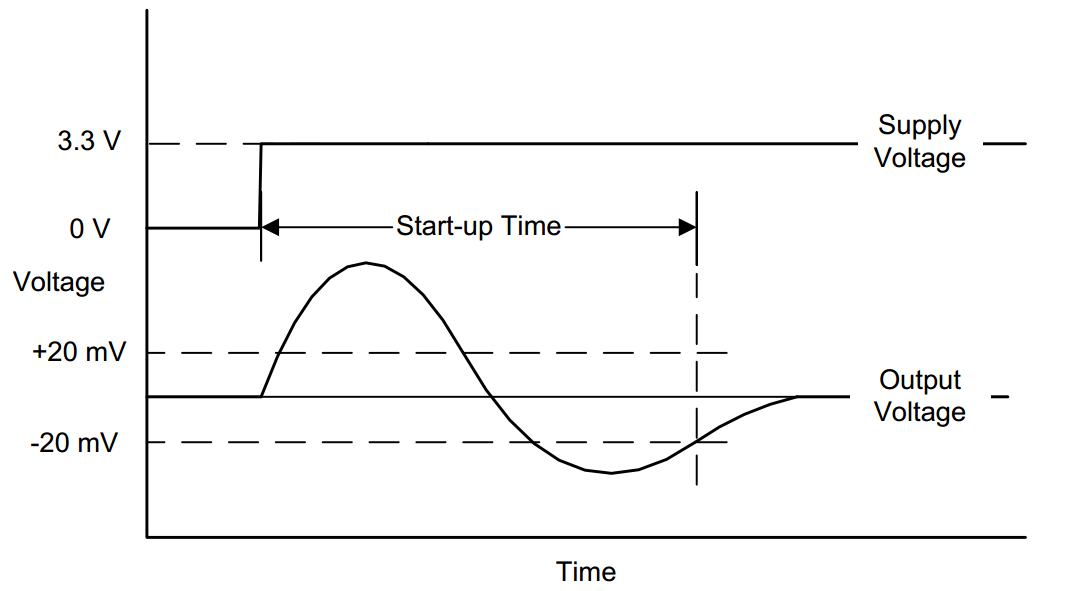##### Figure 6. Image courtesy of Texas Instruments.

As shown in Figure 6, the start-up time is defined as the time duration it takes the device output to settle to within 20 mV of its nominal value after the power is connected.

### Output Swing

With a single-supply operational amplifier, the output generally cannot swing to the ground potential. The output of a good single-supply operational amplifier can only get close to the ground potential. There are applications that require a voltage swing from 0 V to the positive power rail. In these applications, we can use the OPA2333P as shown in Figure 7.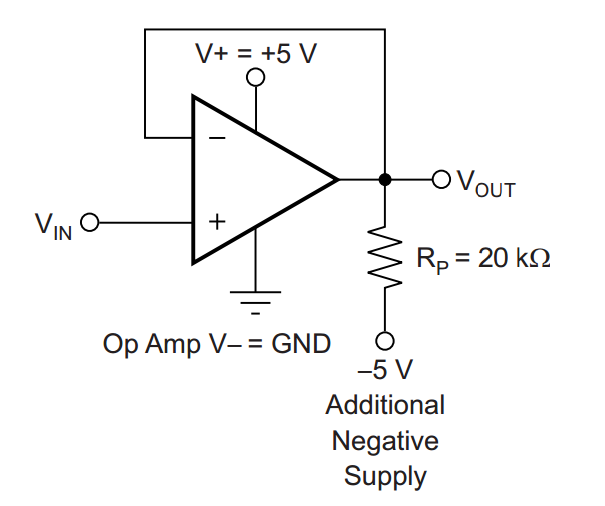##### Figure 7. Image courtesy of Texas Instruments.

With this configuration, the OPA2333P output can swing to voltages even slightly below the ground potential (about -2 mV) without losing its specified accuracy. See the device datasheet for more details.

Also note that, unlike traditional rail-to-rail amplifiers that exhibit crossover distortion, the OPA2333P offers rail-to-rail voltage swing without suffering from crossover distortion associated with the traditional structures.

In this article, we looked at some of the most important features of the OPA2333P from Texas Instruments. Do you have experience with this zero-drift amplifier or other similar parts? Let us know in the comments below.

1 Comment